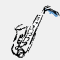Math Is Fun Forum

Discussion about math, puzzles, games and fun.   Useful symbols: ÷ × ½ √ ∞ ≠ ≤ ≥ ≈ ⇒ ± ∈ Δ θ ∴ ∑ ∫  π  -¹ ² ³ °

You are not logged in.

#1 2006-09-29 22:04:20

justlookingforthemoment
ModeratorRegistered: 2005-05-26
Posts: 2,161

Expanding two factors

Expanding two factors

Expand and simplify the following:

1. 4(x + 3)
2. 3(x + 7)
3. 8(x - 2)
4. 5(x - 4)
5. 3x(x + 6)
6. 7x(x + 2)
7. -4x(x + 2)
8. -5x(x + 3)
9. -6x(x - 1)
10. -2x(x - 5)

11. x(2x + 1) + 5(x - 12)
12. 3(x² + 4) - 2(6x - 9)
13. 6x(2x² - x) - 3(x - 7)

14. (x + 1)(x + 3)
15. (x + 2)(x + 7)
16. (x + 4)(x - 2)
17. (x - 8)(x - 3)
18. (2x - 1)(3 - 2)
19. (6x + 2)(2x - 5)
20. (3x - 4)(x - 2)

21. Robin's rug is (2y + 2) metres long and y metres wide. Find the surface area the rug would cover, in terms of y.
22. A plank of wood has a length of (4x + 2)cm and a width of (x + 3)cm. Find the area of the plank, in terms of x.

Offline

#2 2006-11-02 02:29:09

Toast
Real MemberRegistered: 2006-10-08
Posts: 1,321

Offline